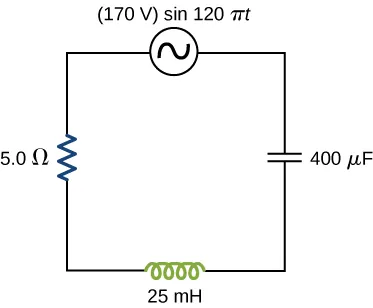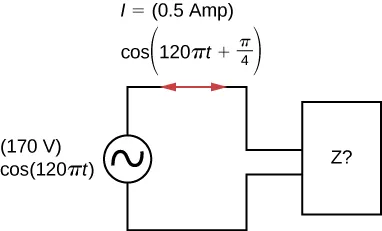University Physics Volume 2

# Problems

### 15.1AC Sources

14.

Write an expression for the output voltage of an ac source that has an amplitude of 12 V and a frequency of 200 Hz.

### 15.2Simple AC Circuits

15.

Calculate the reactance of a $5.0-μF5.0-μF$ capacitor at (a) 60 Hz, (b) 600 Hz, and (c) 6000 Hz.

16.

What is the capacitance of a capacitor whose reactance is $10Ω10Ω$ at 60 Hz?

17.

Calculate the reactance of a 5.0-mH inductor at (a) 60 Hz, (b) 600 Hz, and (c) 6000 Hz.

18.

What is the self-inductance of a coil whose reactance is $10Ω10Ω$ at 60 Hz?

19.

At what frequency is the reactance of a $20-μF20-μF$ capacitor equal to that of a 10-mH inductor?

20.

At 1000 Hz, the reactance of a 5.0-mH inductor is equal to the reactance of a particular capacitor. What is the capacitance of the capacitor?

21.

A $50-Ω50-Ω$ resistor is connected across the emf $v(t)=(160V)sin(120πt)v(t)=(160V)sin(120πt)$. Write an expression for the current through the resistor.

22.

A $25-μF25-μF$ capacitor is connected to an emf given by $v(t)=(160V)sin(120πt)v(t)=(160V)sin(120πt)$. (a) What is the reactance of the capacitor? (b) Write an expression for the current output of the source.

23.

A 100-mH inductor is connected across the emf of the preceding problem. (a) What is the reactance of the inductor? (b) Write an expression for the current through the inductor.

### 15.3RLC Series Circuits with AC

24.

What is the impedance of a series combination of a $50-Ω50-Ω$ resistor, a $5.0-μF5.0-μF$ capacitor, and a $10-μF10-μF$ capacitor at a frequency of 2.0 kHz?

25.

A resistor and capacitor are connected in series across an ac generator. The emf of the generator is given by $v(t)=V0cosωt,v(t)=V0cosωt,$ where $V0=120V,V0=120V,$ $ω=120πrad/s,ω=120πrad/s,$ $R=400ΩR=400Ω$, and $C=4.0μFC=4.0μF$. (a) What is the impedance of the circuit? (b) What is the amplitude of the current through the resistor? (c) Write an expression for the current through the resistor. (d) Write expressions representing the voltages across the resistor and across the capacitor.

26.

A resistor and inductor are connected in series across an ac generator. The emf of the generator is given by $v(t)=V0cosωt,v(t)=V0cosωt,$ where $V0=120VV0=120V$ and $ω=120πrad/s;ω=120πrad/s;$ also, $R=400ΩR=400Ω$ and $L=1.5H.L=1.5H.$ (a) What is the impedance of the circuit? (b) What is the amplitude of the current through the resistor? (c) Write an expression for the current through the resistor. (d) Write expressions representing the voltages across the resistor and across the inductor.

27.

In an RLC series circuit, the voltage amplitude and frequency of the source are 100 V and 500 Hz, respectively, an $R=500Ω,R=500Ω,$ $L=0.20H,L=0.20H,$ and $C=2.0μF.C=2.0μF.$ (a) What is the impedance of the circuit? (b) What is the amplitude of the current from the source? (c) If the emf of the source is given by $v(t)=(100V)sin1000πtv(t)=(100V)sin1000πt$, how does the current vary with time? (d) Repeat the calculations with C changed to $0.20μF.0.20μF.$

28.

An RLC series circuit with $R=600ΩR=600Ω$, $L=30mH,L=30mH,$ and $C=0.050μFC=0.050μF$ is driven by an ac source whose frequency and voltage amplitude are 500 Hz and 50 V, respectively. (a) What is the impedance of the circuit? (b) What is the amplitude of the current in the circuit? (c) What is the phase angle between the emf of the source and the current?

29.

For the circuit shown below, what are (a) the total impedance and (b) the phase angle between the current and the emf? (c) Write an expression for $i(t).i(t).$### 15.4Power in an AC Circuit

30.

The emf of an ac source is given by $v(t)=V0sinωt,v(t)=V0sinωt,$ where $V0=100VV0=100V$ and $ω=200πrad/s.ω=200πrad/s.$ Calculate the average power output of the source if it is connected across (a) a $20-μF20-μF$ capacitor, (b) a 20-mH inductor, and (c) a $50-Ω50-Ω$ resistor.

31.

Calculate the rms currents for an ac source is given by $v(t)=V0sinωt,v(t)=V0sinωt,$ where $V0=100VV0=100V$ and $ω=200πrad/sω=200πrad/s$ when connected across (a) a $20-μF20-μF$ capacitor, (b) a 20-mH inductor, and (c) a $50-Ω50-Ω$ resistor.

32.

A 40-mH inductor is connected to a 60-Hz AC source whose voltage amplitude is 50 V. If an AC voltmeter is placed across the inductor, what does it read?

33.

For an RLC series circuit, the voltage amplitude and frequency of the source are 100 V and 500 Hz, respectively; $R=500ΩR=500Ω$; and $L=0.20HL=0.20H$. Find the average power dissipated in the resistor for the following values for the capacitance: (a) $C=2.0μFC=2.0μF$ and (b) $C=0.20μF.C=0.20μF.$

34.

An ac source of voltage amplitude 10 V delivers electric energy at a rate of 0.80 W when its current output is 2.5 A. What is the phase angle $ϕϕ$ between the emf and the current?

35.

An RLC series circuit has an impedance of $60Ω60Ω$ and a power factor of 0.50, with the voltage lagging the current. (a) Should a capacitor or an inductor be placed in series with the elements to raise the power factor of the circuit? (b) What is the value of the reactance across the inductor that will raise the power factor to unity?

### 15.5Resonance in an AC Circuit

36.

(a) Calculate the resonant angular frequency of an RLC series circuit for which $R=20ΩR=20Ω$, , and $C=4.0μF.C=4.0μF.$ (b) If R is changed to $300Ω,300Ω,$ what happens to the resonant angular frequency?

37.

The resonant frequency of an RLC series circuit is $2.0×103Hz.2.0×103Hz.$ If the self-inductance in the circuit is 5.0 mH, what is the capacitance in the circuit?

38.

(a) What is the resonant frequency of an RLC series circuit with $R=20ΩR=20Ω$, $L=2.0mHL=2.0mH$, and $C=4.0μFC=4.0μF$? (b) What is the impedance of the circuit at resonance?

39.

For an RLC series circuit, $R=100ΩR=100Ω$, $L=150mHL=150mH$, and $C=0.25μF.C=0.25μF.$ (a) If an ac source of variable frequency is connected to the circuit, at what frequency is maximum power dissipated in the resistor? (b) What is the quality factor of the circuit?

40.

An ac source of voltage amplitude 100 V and variable frequency f drives an RLC series circuit with $R=10ΩR=10Ω$, $L=2.0mHL=2.0mH$, and $C=25μF.C=25μF.$ (a) Plot the current through the resistor as a function of the frequency f. (b) Use the plot to determine the resonant frequency of the circuit.

41.

(a) What is the resonant frequency of a resistor, capacitor, and inductor connected in series if $R=100Ω,R=100Ω,$ $L=2.0HL=2.0H$, and $C=5.0μFC=5.0μF$? (b) If this combination is connected to a 100-V source operating at the resonant frequency, what is the power output of the source? (c) What is the Q of the circuit? (d) What is the bandwidth of the circuit?

42.

Suppose a coil has a self-inductance of 20.0 H and a resistance of $200Ω200Ω$. What (a) capacitance and (b) resistance must be connected in series with the coil to produce a circuit that has a resonant frequency of 100 Hz and a Q of 10?

43.

An ac generator is connected to a device whose internal circuits are not known. We only know current and voltage outside the device, as shown below. Based on the information given, what can you infer about the electrical nature of the device and its power usage?### 15.6Transformers

44.

A step-up transformer is designed so that the output of its secondary winding is 2000 V (rms) when the primary winding is connected to a 110-V (rms) line voltage. (a) If there are 100 turns in the primary winding, how many turns are there in the secondary winding? (b) If a resistor connected across the secondary winding draws an rms current of 0.75 A, what is the current in the primary winding?

45.

A step-up transformer connected to a 110-V line is used to supply a hydrogen-gas discharge tube with 5.0 kV (rms). The tube dissipates 75 W of power. (a) What is the ratio of the number of turns in the secondary winding to the number of turns in the primary winding? (b) What are the rms currents in the primary and secondary windings? (c) What is the effective resistance seen by the 110-V source?

46.

An ac source of emf delivers 5.0 mW of power at an rms current of 2.0 mA when it is connected to the primary coil of a transformer. The rms voltage across the secondary coil is 20 V. (a) What are the voltage across the primary coil and the current through the secondary coil? (b) What is the ratio of secondary to primary turns for the transformer?

47.

A transformer is used to step down 110 V from a wall socket to 9.0 V for a radio. (a) If the primary winding has 500 turns, how many turns does the secondary winding have? (b) If the radio operates at a current of 500 mA, what is the current through the primary winding?

48.

A transformer is used to supply a 12-V model train with power from a 110-V wall plug. The train operates at 50 W of power. (a) What is the rms current in the secondary coil of the transformer? (b) What is the rms current in the primary coil? (c) What is the ratio of the number of primary to secondary turns? (d) What is the resistance of the train? (e) What is the resistance seen by the 110-V source?

Order a print copy

As an Amazon Associate we earn from qualifying purchases.